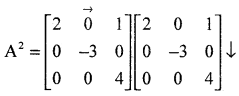# 2nd PUC Maths Question Bank Chapter 3 Matrices

Students can Download 2nd PUC Maths Chapter 3 Matrices Questions and Answers, Notes Pdf, 2nd PUC Maths Question Bank with Answers helps you to revise the complete Karnataka State Board Syllabus and to clear all their doubts, score well in final exams.

## Karnataka 2nd PUC Maths Question Bank Chapter 3 Matrices

### 2nd PUC Maths Matrices One Mark Questions and Answers

Question 1.
Define a diagonal matrix. (June 2014)
A square matrix is said to be a diagonal matrix if all its non diagonal elements are zero.

Question 2.
Define a scalar matrix. (March 2014)
A diagonal matrix is said to be a scalar matrix if its diagonal elements are equal

Question 3.
Define a unit matrix.
A square matrix in which elements in the principal diagonal are all 1 and rest are all zero is called an identity matrix or a unit matrix.Question 4.
Define a symmetric matrix.
A square matrix A is said to be symmetric if A1 = A

Question 5.
Define a skew-symmetric matrix.
A square matrix A is said to be skew-symmetric if A1 = – A

Question 6.
Construct a 2 × 3 whose elements are given by aij =|i – j|.
a11 = |1 – 1| = |0| = 0
a12 = |1 – 2| = |-1| = 1
a13 = |1 – 3| =|-2| = 2
a21 = |2 – 1| = |1| = 1
a22 = |2 – 2| = |0|= 0
a23 = |2 – 3| = |-1| = 1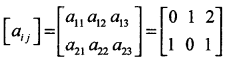Question 7.
Construct a 3 × 2 matrix whose elements are given by aij = $$\frac { 1 }{ 2 }$$|i – 3j|.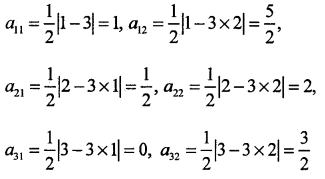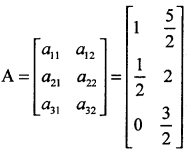Question 8.
In a matrix write.
(i) the order of the matrix,
(ii) the number of elements,
(iii)write the lements a13, a21, a33, a24, a23.
In the given matrix number of rows is 3 and number of columns is 4.
∴ the order of the matrix is 3 × 4 and the number of elements is 3 × 4 = 12.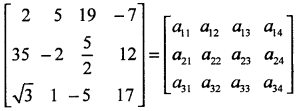On comparing the corresponding elements we get
a13 = 19: a21 = 35: a33 = -5: a24 = 12: a23 = $$\frac { 5 }{ 2 }$$

Question 9.
Construct a 2 × 2 matrix, A = [aij], whose elements are given by:
(i) aij = $$\frac{(i+j)^{2}}{2}$$
(ii) aij = $$\frac{i}{j}$$
(iii) aij = $$\frac{(i+2 j)^{2}}{2}$$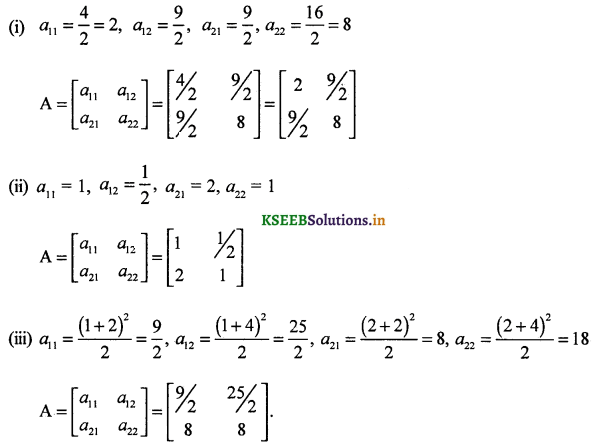Question 10.
What is the number of the possible square matrices for order 2 with each entry 0 or 1
As order of 2 × 2 matrix contains 4 elements. Each element can be selected in 2 ways (it can be either 0 or 1). Hence, all the four entries can be chosen in 24 = 16.

Question 11.
If a matrix has 18 elements what are the possible orders it can have?
1 × 18, 2 × 9, 3 × 6, 6 × 3, 9 × 2,18 × 1.Question 12.
If a matrix has 5 elements what are the possible orders it can have?
1 × 5, 5 × 1.

Question 13.
Find the transpose of the matrix: $$\left[ \begin{matrix} 5 \\ \frac { 1 }{ 2 } \\ -1 \end{matrix} \right]$$
$$\left[ \begin{matrix} 5 & \frac { 1 }{ 2 } & -1 \end{matrix} \right]$$

Question 14.
Construct a 2 × 3 matrix whose elements are given by aij = |i – j|
aij = |i – j| 2 × 3 matrixQuestion 15.
What is the number of the possible square matrices for order 3 with each entry 0 or 1? (March 2014)
The number of possible square matrices with order 3 and with each entry 0 or 1 is 512.

Question 16.
Construct a 2 × 2 matrix A = |aij| whose elements are given by a- = 2i + jQuestion 17.
Construct a 2 × 2 matrix A = |aij| whose elements are given by aij = $$\frac { 1 }{ 2 }$$|-3i + j|### 2nd PUC Maths Matrices Three Marks Questions with Answers

Question 1.
Find the value of a, b, c and d from the equation: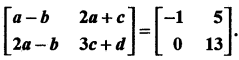a – b= – 1 (i)
2a + c = 5 (ii)
2a – b = 0 (iii)
3c + d = 13 (iv)
2 a = b (from iii) substituting in (i) a – 2a = -1 ⇒ a = 1
On simplifying we get b = 2, c = 3 and d = 4.

Question 2.Question 3.
Find X and Y if (i) X + Y = $$\left[ \begin{matrix} 7 & 0 \\ 2 & 5 \end{matrix} \right]$$ and X – Y = $$\left[ \begin{matrix} 3 & 0 \\ 3 & 3 \end{matrix} \right]$$
(ii) 2X + 3Y = $$\left[ \begin{matrix} 2 & 3 \\ 4 & 0 \end{matrix} \right]$$ and 3X + 2Y = $$\left[ \begin{matrix} 2 & -2 \\ -1 & 5 \end{matrix} \right]$$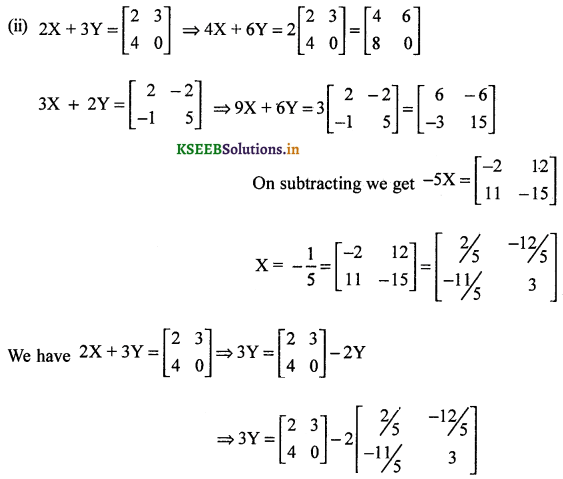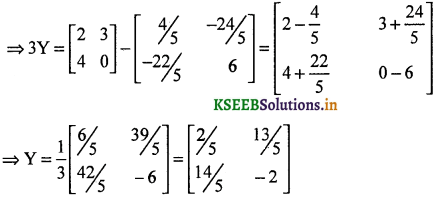Question 4.
Express $$\left[ \begin{matrix} 1 & 2 \\ 3 & 4 \end{matrix} \right]$$ as sum of a symmetric and skew symmetric matrices.
Let A = $$\left[ \begin{matrix} 1 & 2 \\ 3 & 4 \end{matrix} \right]$$, then A = p + QClearly P = P’ and Q = – Q P ¡s a symmetric matrix and Q is a skew – symmetric matrix and A = P + Q.

Question 5.
By using the elementary transformation, find the inverse of the following matrices.
(i) $$\left[ \begin{matrix} 1 & 3 \\ 2 & 7 \end{matrix} \right]$$
Let A = $$\left[ \begin{matrix} 1 & 3 \\ 2 & 7 \end{matrix} \right]$$ we know that A = IA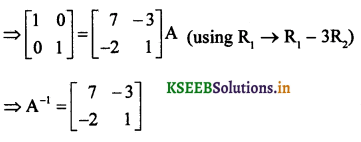(ii) $$\left[ \begin{matrix} 2 & 3 \\ 5 & 7 \end{matrix} \right]$$
Let $$\left[ \begin{matrix} 2 & 3 \\ 5 & 7 \end{matrix} \right]$$ we know that A = IA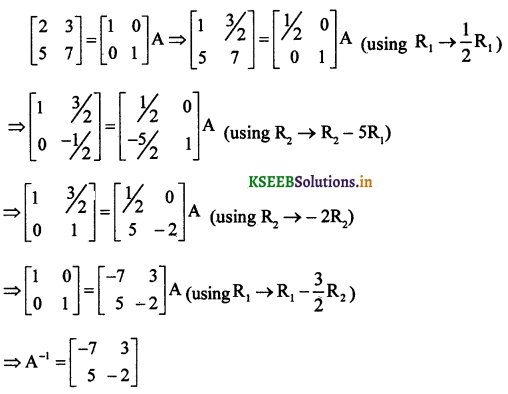Question 6.
If A = $$\left[ \begin{matrix} -2 \\ 4 \\ 5 \end{matrix} \right]$$, B = $$\left[ \begin{matrix} 1 & 3 & -6 \end{matrix} \right]$$, verify that (AB)’ = B’A’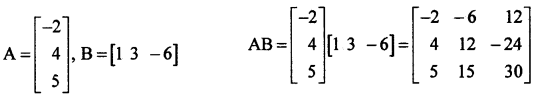Question 7.
By using the elementary transformation, find the inverse of the matrix A = $$\left[ \begin{matrix} 1 & -2 \\ 2 & 1 \end{matrix} \right]$$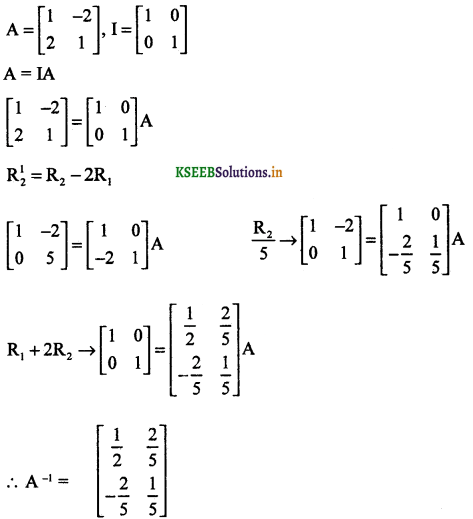Question 8.
If A and B are square matrices of the same order, then shQw that (AB)-1 = B-1A-1 (March 2015)
To prove (AB)-1 = B-1A-1
We know that (AB)(AB)-1 = I
Pre multiply by (A)-1
A-1 [(AB)(AB)-1] = IA-1 (A-1A)B(AB)-1 = A-1
IB(AB)-1 = A-1 B(AB)-1 = A-1
Pre multiply by B-1
B-1B(AB)-1 = B-1A-1 I(AB)-1 = B-1A-1
(AB)-1 = B’A’
Hence proved.Question 9.
Express matrix A = $$\left[ \begin{matrix} 1 & 2 \\ 2 & -1 \end{matrix} \right]$$ as the sum of a symmetric and skew-symmetric matrix.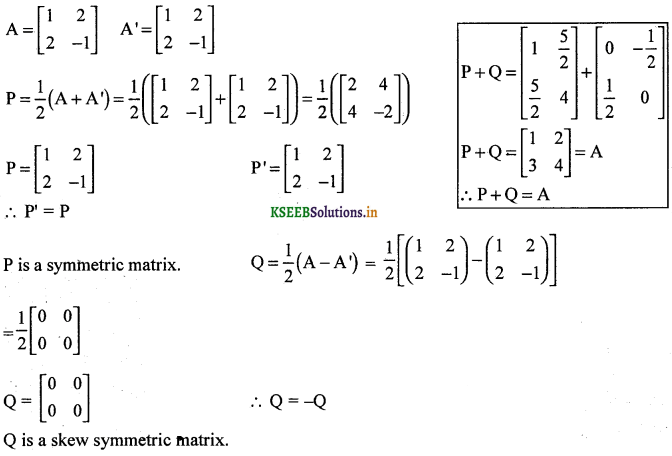Question 10.
Find the value of x and y in $$\left[ \begin{matrix} x+2y & 2 \\ 4 & x+y \end{matrix} \right] -\left[ \begin{matrix} 3 & 2 \\ 4 & 1 \end{matrix} \right]$$ = 0 where 0 is a null matrix.x + 2y – 3 = 0
x + y – 1 = 0
x + 2y = 3 …………… (1)
x + y = 1 …………. (2)
subtracting (2) from (1)
y = 2
Substituting y = 2 in eq (2)
x + 2 = 1
x = 1 – 2
x = – 1Question 11.
Find the value of x and y = $$\left[ \begin{matrix} x+y & 3 \\ x-y & -6 \end{matrix} \right] =\left[ \begin{matrix} 2 & 3 \\ 4 & -6 \end{matrix} \right]$$
$$\left[ \begin{matrix} x+y & 3 \\ x-y & -6 \end{matrix} \right] =\left[ \begin{matrix} 2 & 3 \\ 4 & -6 \end{matrix} \right]$$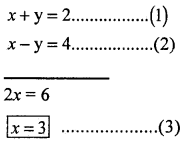Substituting eqn. (3) in eqn. (2)
3x + y = 2
y = 2 – 3
y = -15 + z = 5
z = 0
∴ x = 4, y = 2, z = 0
or
x = 2, y = 4, z = 0

Question 12.
For any square matriz A with real numbers, prove that A + A’ is a symmetric and A – A’ ¡s a skew symmetric. (June 2014)
Let B = A + A’
B’ = (A + A’)’
⇒ B’ = A’ + (A’)’ = A’ + A = A + A’ = B
∴ B = A + A’ is a symmetric matrix.
Let C = A – A’
⇒ C’ = (A – A’)’ = A’ – (A’)’ = A’ – A = – (A – A’) = – C.
∴ C = A – A’ is a skew symmetric matrix.

Question 13.
If $$\left[ \begin{matrix} 3 & -2 \\ 4 & -2 \end{matrix} \right]$$, find k so that A2 = kA – 2I.
A2 = kA – 2IQuestion 14.
A trust fund has Rs. 30,000 that must be invested in two different types of bonds. The first bond pays 5% interest per year, and the second bond pays 7% interest per year. Using matrix multiplication, determine how to divide Rs. 30,000 among th two types of bonds. If the trust fund must obtain an annual total interest of Rs 1800.
Let the amount invested in first type of bonds be Rs x. then that invested in second type of
bonds will be Rs (30,000 – x)
According to the given condition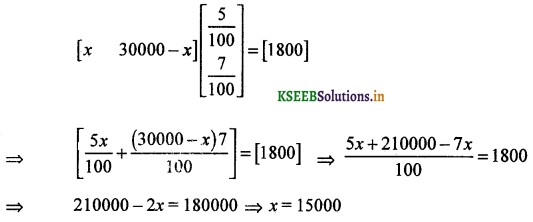∴ The amounts invested in two types of bonds are Rs. 15,000 and 30,000 – 15,000 = 15,000### 2nd PUC Maths Matrices Five Marks Questions and Answers

Question 1.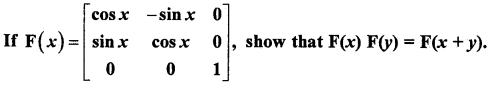Question 2.
Find A2 – 5A + 6I if A = $$\left[ \begin{matrix} 2 & 0 & 1 \\ 2 & 1 & 3 \\ 1 & -1 & 0 \end{matrix} \right]$$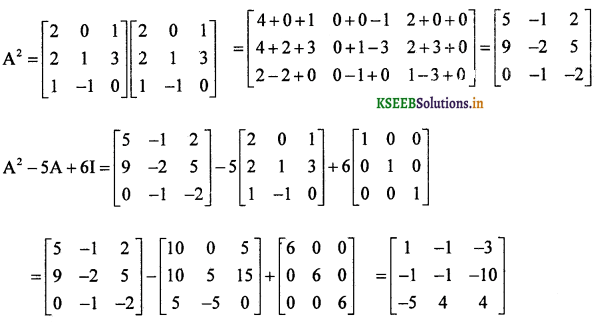Question 3.
If A = $$\left[ \begin{matrix} 1 & 0 & 2 \\ 0 & 2 & 1 \\ 2 & 0 & 3 \end{matrix} \right]$$, prove that A3 – 6A2 + 7A + 2I = 0Question 4.
If A = $$\left[ \begin{matrix} 1 & -1 \\ 2 & 3 \end{matrix} \right]$$, B = $$\left[ \begin{matrix} 1 & 3 \\ -1 & 4 \end{matrix} \right]$$ and C = $$\left[ \begin{matrix} 2 & -2 \\ 3 & 0 \end{matrix} \right]$$ verify that A(BC) = (AB)C.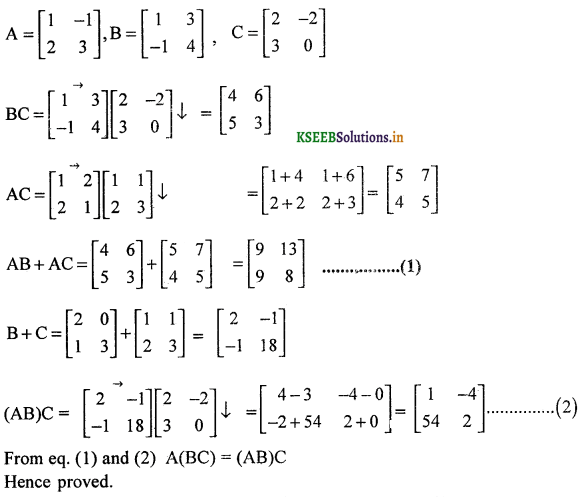Question 5.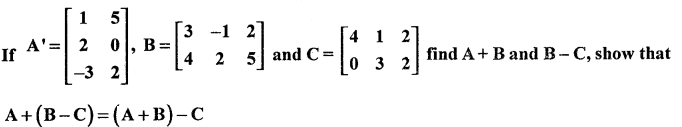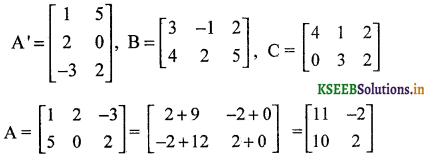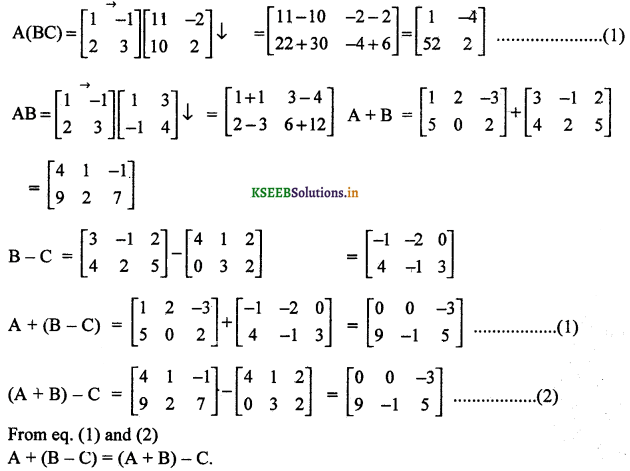Question 6.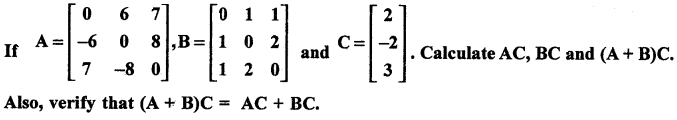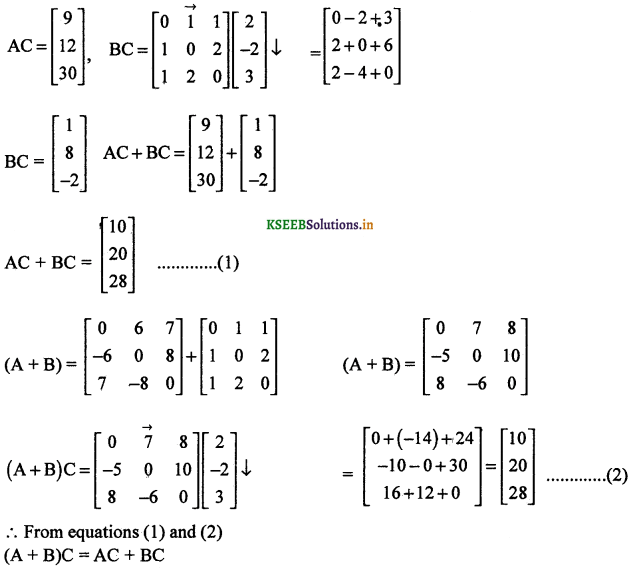Question 7.
If A = $$\left[ \begin{matrix} -2 \\ 4 \\ 5 \end{matrix} \right]$$, B = $$\left( \begin{matrix} 1 & 3 & -6 \end{matrix} \right)$$ verify that (AB)’ = B’A’ (June 2014)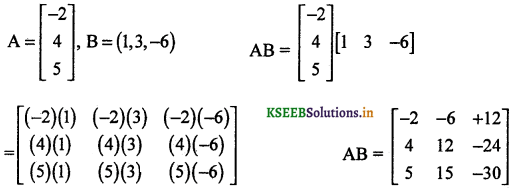Question 8.
If A = $$\left[ \begin{matrix} 2 & 0 & 1 \\ 0 & -3 & 0 \\ 0 & 0 & 4 \end{matrix} \right]$$, verify
A3 – 3A2 – 10A + 24I = 0 where 0 is zero matrix of order 3 × 3
A = $$\left[ \begin{matrix} 2 & 0 & 1 \\ 0 & -3 & 0 \\ 0 & 0 & 4 \end{matrix} \right]$$